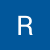# Generating global graph structure using local 1D scalar embeddings

Source: Deep Learning on Medium

Random walks have been used to capture topological features (i.e. patterns of edges) of graphs since DeepWalk. It is done to obtain the graph embeddings from the samples taken along the random walks. The embeddings are a set of multivariate function on each of the nodes, S = {f(1),…, f(i),…,f(N)}. The typical number of dimensions of an embedding is in the range of [100,1000].

We managed to theoretically derive a set of 1-dimensional node embeddings that reflects the topological features of graphs, as opposed to the O(100) dimensional embeddings learned by machine learning algorithms. Under mild assumptions, the set of such 1-dimensional embeddings exists and is unique for each graph.

Based on this theoretically derived embedding, we propose a method to generate smaller topological equivalent graphs for machine learning models to train from, rather than feeding subgraphs sampled from random walks on a much larger graph as usually done in the literature. Our generated graphs are topologically similar, but is not necessarily a subgraph in the larger graph provided by the data.

We compared our approach against a state-of-the-art graph generation algorithm, GraphGAN, and observed that our approach requires 3 to 4 times shorter training time, while significantly outperforming GraphGAN and other state-of-the-art methods in predictive accuracy. The results indicates that the theoretical model captures the graph topology better than GraphGAN.

The task of link prediction on a graph is as follows. Suppose that the dataset is a large graph, with distinct topologies, or patterns of edges, repeating throughout the graph. The task is to predict edges in any node pairs in the graph. To create training batches for topological data, a typical method is to generate subgraphs centered on a node. The set of all node pairs within each subgraph is used for training. This process is repeated for all nodes in the graph.

Because the generated node pairs in a batch does not contain topological information by themselves, the model must rely on the subgraph generation method to provide such information.

### Experiments

On sparse networks, our theoretical embedding takes on a closed form. We show below with this approximation that our approach outperforms GraphGAN on two real datasets.

To obtain our model, we simply replace all subgraph generation procedures in GraphGAN with our approach using the scalar embedding.

#### Datasets

We tested on a private social network dataset and the astrophysics citation network. The social network dataset has about 134,000 nodes and 357,000 edges. The astrophysics citation network is much denser, it consists of 17,000 nodes and 355,00 edges. A visualization of our private dataset is shown below.

To form the training and validation data set, we hide 10% of the edges in the graph as positive samples and generate unlinked pairs as negative samples for the validation set. The remaining 90% of the edges is used for training.

#### Results

The below figure shows the validation accuracy versus time spent for the experiment on the social network.